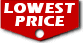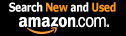# Introduction to Graph Theory (Dover Books on Mathematics) / Richard J. Trudeau

List Price: \$16.95
Our Price: \$12.19For Bulk orders
Quantity

Used Book Price:
/ Richard J. Trudeau
Publisher: Dover Publications
Availability:In Stock.
Sales Rank: 27549
 ISBN-10: 0486678709ISBN-13: 9780486678702

Similar Books
 Introduction to Topology: Third Edition (Dover Books on Mathematics) An Introduction to Information Theory: Symbols, Signals and Noise (Dover Books on Mathematics) Number Theory (Dover Books on Mathematics) Probability Theory: A Concise Course (Dover Books on Mathematics) Linear Algebra (Dover Books on Mathematics) Introductory Discrete Mathematics (Dover Books on Computer Science) Ordinary Differential Equations (Dover Books on Mathematics) A First Course in Graph Theory (Dover Books on Mathematics) Fourier Series (Dover Books on Mathematics) A Book of Abstract Algebra: Second Edition (Dover Books on Mathematics)

A stimulating excursion into pure mathematics aimed at "the mathematically traumatized," but great fun for mathematical hobbyists and serious mathematicians as well. Requiring only high school algebra as mathematical background, the book leads the reader from simple graphs through planar graphs, Euler's formula, Platonic graphs, coloring, the genus of a graph, Euler walks, Hamilton walks, and a discussion of The Seven Bridges of Konigsberg. Exercises are included at the end of each chapter. "The topics are so well motivated, the exposition so lucid and delightful, that the book's appeal should be virtually universal . . . Every library should have several copies" � Choice. 1976 edition.

Now you can buy Books online in USA,UK, India and more than 100 countries.
*Terms and Conditions apply
Disclaimer: All product data on this page belongs to.
No guarantees are made as to accuracy of prices and information.